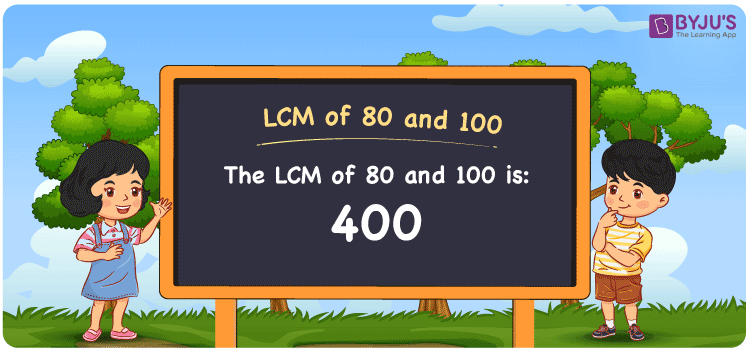# LCM of 80 and 100

LCM of 80 and 100 is 400. The smallest number among all common multiples of 80 and 100 is the LCM of 80 and 100. (80, 160, 240, 320, 400, 480, etc.) and (100, 200, 300, 400, 500, 600, etc.) are the first few multiples of 80 and 100, respectively. To find the LCM of 80 and 100, there are three typical methods: division, prime factorization, and listing multiples. In Maths, the LCM of any two numbers is the value which is evenly divisible by the given two numbers.

Also read: Least common multiple

## What is LCM of 80 and 100?

The answer to this question is 400. The LCM of 80 and 100 using various methods is shown in this article for your reference. The LCM of two non-zero integers, 80 and 100, is the smallest positive integer 400 which is divisible by both 80 and 100 with no remainder.## How to Find LCM of 80 and 100?

LCM of 80 and 100 can be found using three methods:

• Prime Factorisation
• Division method
• Listing the multiples

### LCM of 80 and 100 Using Prime Factorisation Method

The prime factorisation of 80 and 100, respectively, is given by:

80 = (2 × 2 × 2 × 2 × 5) = 24 × 51 and

100 = (2 × 2 × 5 × 5) = 22 × 52

LCM (80, 100) = 400

### LCM of 80 and 100 Using Division Method

We’ll divide the numbers (80, 100) by their prime factors to get the LCM of 80 and 100 using the division method (preferably common). The LCM of 80 and 100 is calculated by multiplying these divisors.

 2 80 100 2 40 50 2 20 25 2 10 25 5 5 25 5 1 5 x 1 1

No further division can be done.

Hence, LCM (80, 100) = 400

### LCM of 80 and 100 Using Listing the Multiples

To calculate the LCM of 80 and 100 by listing out the common multiples, list the multiples as shown below

 Multiples of 80 Multiples of 100 80 100 160 200 240 300 320 400 400 500

The smallest common multiple of 80 and 100 is 400.

Therefore LCM (80, 100) = 400

## Video Lesson on Applications of LCM## LCM of 80 and 100 Solved Example

The product of two numbers is 8000. If their GCD is 20, what is their LCM?

Solution:

Given: GCD = 20

product of numbers = 8000

∵ LCM × GCD = product of numbers

⇒ LCM = Product/GCD = 8000/20

Therefore, the LCM is 400.

The probable combination for the given case is LCM(80, 100) = 400.

## Frequently Asked Questions on LCM of 80 and 100

### What is the LCM of 80 and 100?

The LCM of 80 and 100 is 400. To find the least common multiple of 80 and 100, we need to find the multiples of 80 and 100 (multiples of 80 = 80, 160, 240, 320 . . . . 400; multiples of 100 = 100, 200, 300, 400) and choose the smallest multiple that is exactly divisible by 80 and 100, i.e., 400.

### List the methods used to find the LCM of 80 and 100.

The methods used to find the LCM of 80 and 100 are the Prime Factorization Method, Division Method and Listing multiples.

### What is the Least Perfect Square Divisible by 80 and 100?

The least number divisible by 80 and 100 = LCM(80, 100)
LCM of 80 and 100 = 2 × 2 × 2 × 2 × 5 × 5 [No incomplete pair] ⇒ Least perfect square divisible by 80 and 100 = 400 [Square root of 400 = √400 = ±20] Therefore, 400 is the required number.

### What is the Relation Between GCF and LCM of 80, 100?

The following equation can be used to express the relation between GCF and LCM of 80 and 100, i.e. GCF × LCM = 80 × 100.

### If the LCM of 100 and 80 is 400, Find its GCF.

LCM(100, 80) × GCF(100, 80) = 100 × 80
Since the LCM of 100 and 80 = 400
⇒ 400 × GCF(100, 80) = 8000
Therefore, the GCF (greatest common factor) = 8000/400 = 20.